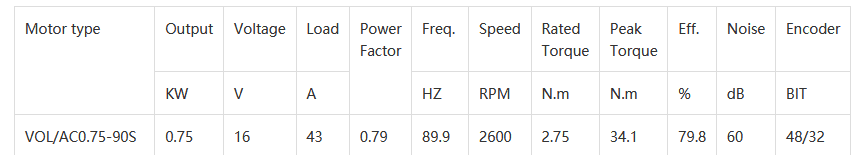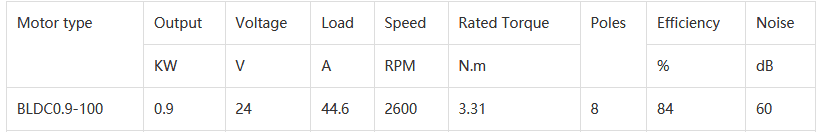# Understanding the Parameters of Synchronous Motors

PhysicsTest
TL;DR Summary
I want to understand the parameters of the synchronous motors.
I want to understand the parameters of the synchronous motor

Rated Power -> This is the maximum power the motor can deliver -> Is it correct? I can operate the motor for any power less than this?

Rated Torque -> This is the maximum torque beyond which the motor cannot produce?

Rated speed -> This is the maximum speed the motor can run upto?

Rated Voltage -> This is the voltage at which the motor can run?

Rated Current -> The motor should not be operated beyond this current? Can i derive this if i know the rated voltage and rated power?

Is the understanding of the above parameters correct? If i assume that the motor is driven by an inverter.

Staff Emeritus
Most ratingsare as you say, the maximum.

Summary:: I want to understand the parameters of the synchronous motors.

Rated speed -> This is the maximum speed the motor can run upto?
Synchronous machines run at synchronous speed. You can't vary the speed.

Summary:: I want to understand the parameters of the synchronous motors.

Rated Current -> The motor should not be operated beyond this current? Can i derive this if i know the rated voltage and rated power?
Only is reactive power is zero. Otherwise, you need voltage and volt amps.

Do you understand reactive (imaginary) power?

•PhysicsTest
Staff Emeritus
I keep forgetting variable frequency AC supplies. If you have one of those, you can vary the speed, and rated speed is maximum speed.

•PhysicsTest
IMO - "rated" typically implies continuous duty. Maximums could be something completely different.

In EVs for example - the operation will take the motor to Zero Speed (RPM) and still have some significant torque. Short term this can be the rated torque, but this may not be continuous rated torque ay zero speed due to localized heating.

Also in specific applications or specific manufacturers have a nominal rating or operating point that they use to help their nomenclature as well.

•cnh1995 and PhysicsTest
Staff Emeritus
IMO - "rated" typically implies continuous duty. Maximums could be something completely different.
Those words are not an exact science. Yes you can exceed rated parameters for short times. Often, thermal effects dominate. But a beginner can start with the basic definition and let nuances come later.

Sometimes, manufacturers provide both numbers. Aircraft have a design cruising speed (rated) and a ##V_{NE}## Never Exceed speed. Above ##V_{NE}##, the wings may break off.

•PhysicsTest
I was referring explicitly to the OP's query regarding Sync Motors- even in an EV the EV's spec will tend to be the MAX power, but that will be limited by the controller, not the physical limits of the device.

•PhysicsTest
PhysicsTest
Only is reactive power is zero. Otherwise, you need voltage and volt amps.
Do you understand reactive (imaginary) power?
Yes i understand it, the reactive power which cannot be used to do useful work, it is stored in inductor and capacitor and then released. But i have seen most manufacturers using KW instead of KVA, then i am not sure how to find the total current to be supplied.
I am still confused with the discussion of the rated parameters. Is there a standard followed or document i can refer to?
Meanwhile i tried to decode the motor parameters from the following link volcano motors

Variable speed AC motorsFrom the above table i understand the O/p power is = 0.75KW. There is no mention of KVA, why? How do i calculate? Is it KVA = 16*43 KVA = 688KVA
I/p power = V*I * Power factor = 16*43 * 0.79 = 543 W = 0.543 KW. I observe the input power is less than the output power of 0.75KW, why?
The other calculation of o/p power is = Torque * Speed (rad/s) = 2.75 * 2600*2 * PI/60 = 748W = 0.748KW. This is matching.
Efficiency = Output / Input , the output is greater than the input, the efficiency is greater than 100%, how is it possible? What is the mistake.

Staff Emeritus
There is no mention of KVA, why?
Power factor is another way to express the same thing, avoiding the need to deal with KVA or VARs.

Good for you for spotting an erroneous >1 efficiency. We either have a misinterpretation, or an error.

My guess is an error by whoever made that nameplate. They might have started with V and P and multiplied by .79 to calculate A where they should have divided. If so, then the amp draw could be 59 A rather than 43. 16*59 * 0.79 = 746.

PhysicsTest
My guess is an error by whoever made that nameplate. They might have started with V and P and multiplied by .79 to calculate A where they should have divided. If so, then the amp draw could be 59 A rather than 43. 16*59 * 0.79 = 746.
The output power is correct using the formula P = Torque * Speed = ##2.75 * 2600*2 * \pi/60 = 748W = 0.748KW.## The efficiency as per the table is 79.8. Hence the input power should be
efficiency= ##748*100/\text{Input power} = 79.8 ##
Input power = ##748*100/79.8 = 937.3 KW##. If i assume the current calculation is wrong then the current is
##V*I*\cos\theta = 937.3 ##
##I = \frac{937.3} {16*0.79} = 74.15 A##

PhysicsTest
I verified another table in the same link Volcano motors for the permanent magnet brushless motorI/p power = 24*44.6 = 1070.4 KW
O/p Power = 2600*3.31*2*PI/60 = 0.9KW
Efficiency = 900*100/1070 = 84% and it matches with the values specified in the table. But my major confusion is there is no power factor mentioned or KVA. Generally any motor contains the inductor and resistance, so why the information is not mentioned?